# 20 points) Consider the periodic signal z(t) shown in the Figure below: X(t) 3 2 N....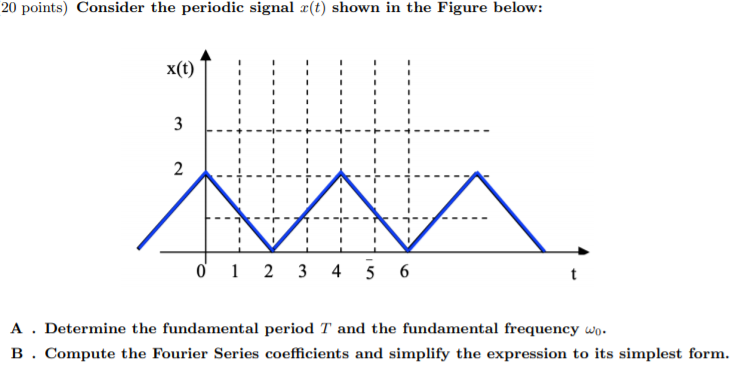20 points) Consider the periodic signal z(t) shown in the Figure below: X(t) 3 2 N. 0 1 2 3 4 5 6 A . Determine the fundamental period T and the fundamental frequency wo. B. Compute the Fourier Series coefficients and simplify the expression to its simplest form.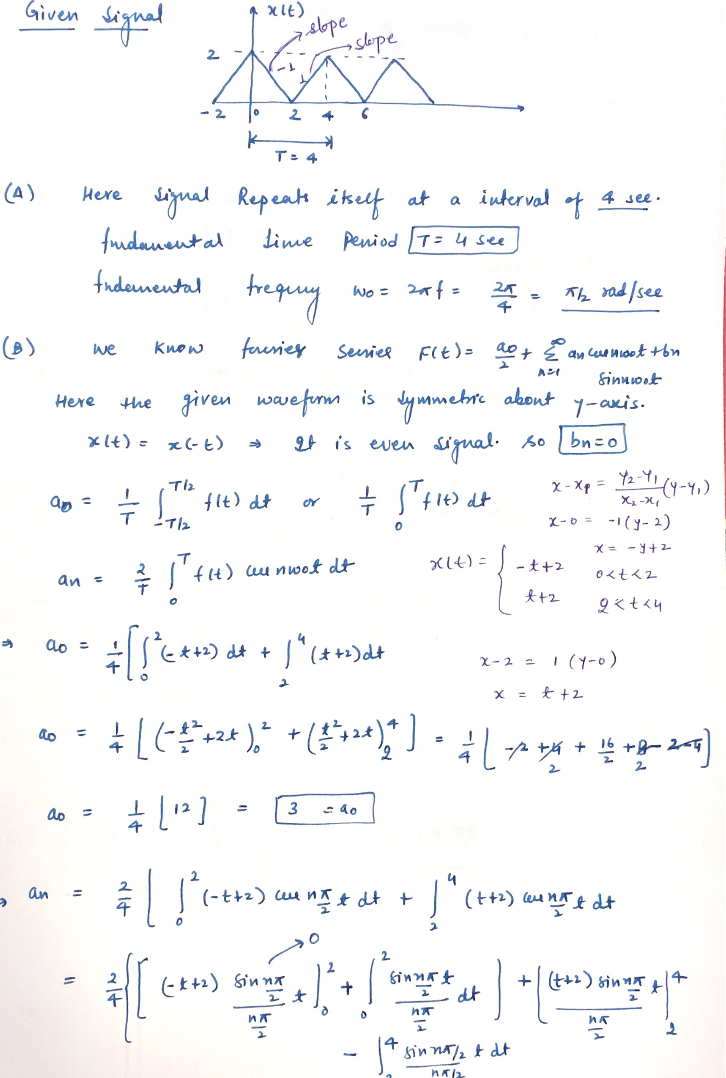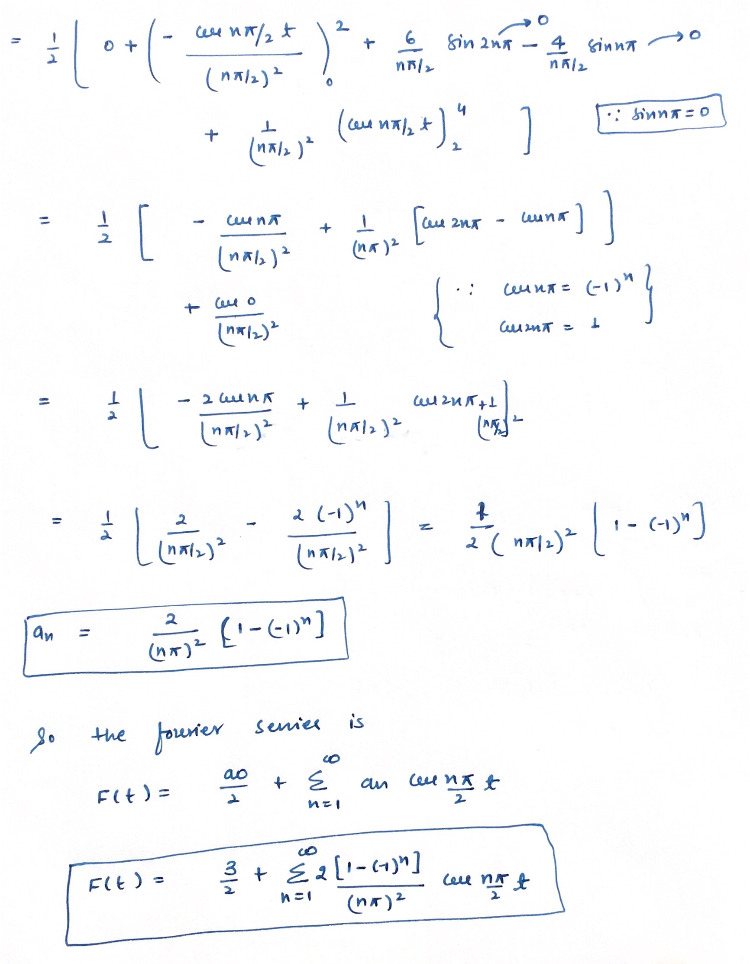Here I am uploading the solution if you have any doubt you can leave the comment.

##### Add Answer of: 20 points) Consider the periodic signal z(t) shown in the Figure below: X(t) 3 2 N....
Similar Homework Help Questions
• ### 4. (20 points) Consider the periodic signal r(t) shown in the Figure below: x(t) 3 2...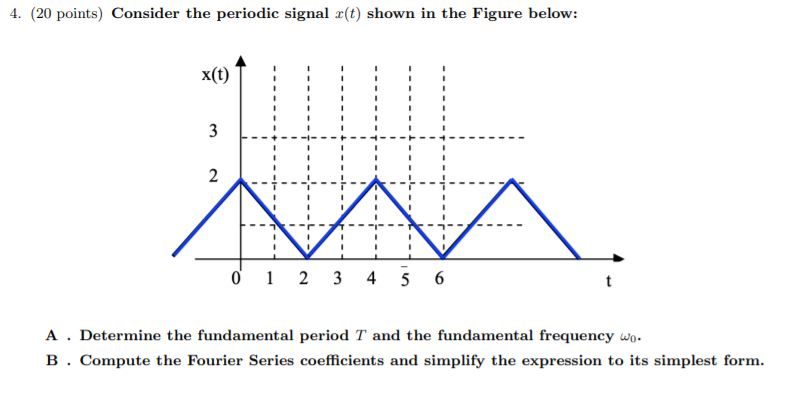4. (20 points) Consider the periodic signal r(t) shown in the Figure below: x(t) 3 2 N VAA 0 1 2 3 4 5 6 A . Determine the fundamental period T and the fundamental frequency wo. B. Compute the Fourier Series coefficients and simplify the expression to its simplest form.

• ### 4. (20 points) Consider the periodic signal z(t) shown in the Figure below: x(t) 1 1...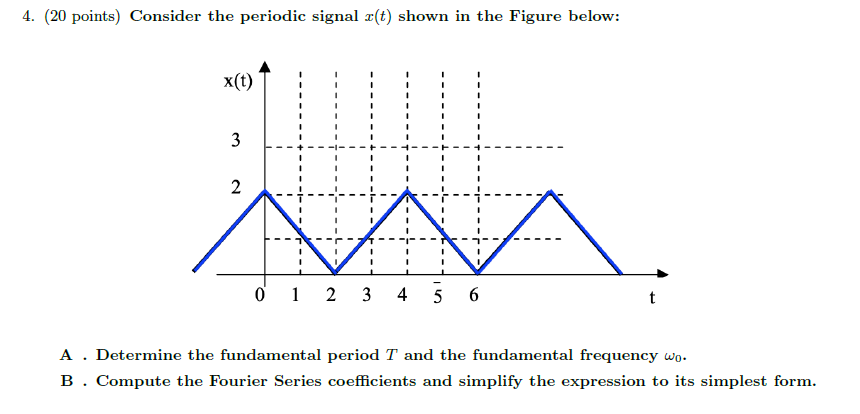4. (20 points) Consider the periodic signal z(t) shown in the Figure below: x(t) 1 1 3 2 - - 1 1 0 1 2 3 4 5 6 t A . Determine the fundamental period T and the fundamental frequency wo. B. Compute the Fourier Series coefficients and simplify the expression to its simplest form.

• ### Write very clear please 4. (20 points) Consider the periodic signal z(t) shown in the Figure...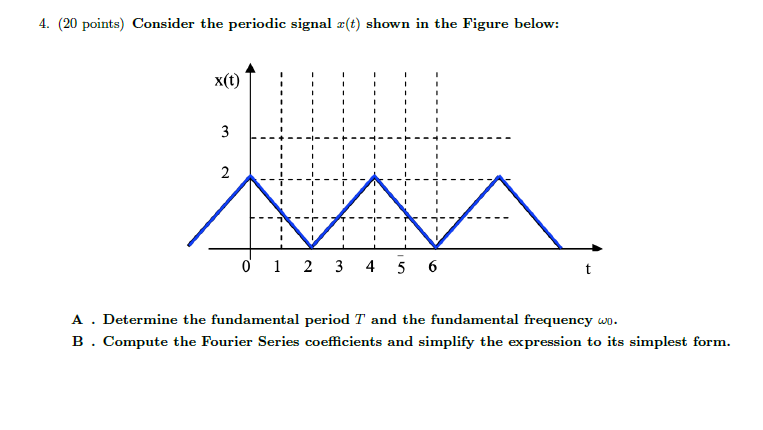Write very clear please 4. (20 points) Consider the periodic signal z(t) shown in the Figure below: 1 x(t) 1 - ! ! 1 3 2 VA 0 1 2 3 4 5 6 t A . Determine the fundamental period T and the fundamental frequency wo. B. Compute the Fourier Series coefficients and simplify the expression to its simplest form.

• ### Consider the periodic signal x(t) shown in the Figure below: x(t) . 3 2 0 1...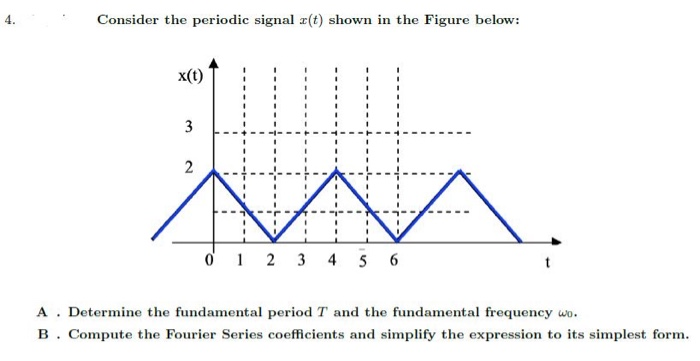Consider the periodic signal x(t) shown in the Figure below: x(t) . 3 2 0 1 2 3 4 5 6 t A. Determine the fundamental period T and the fundamental frequency wo. B. Compute the Fourier Series coefficients and simplify the expression to its simplest form.

• ### A periodic signal x(t) is shown below. We want to find the Fourier Series representation for...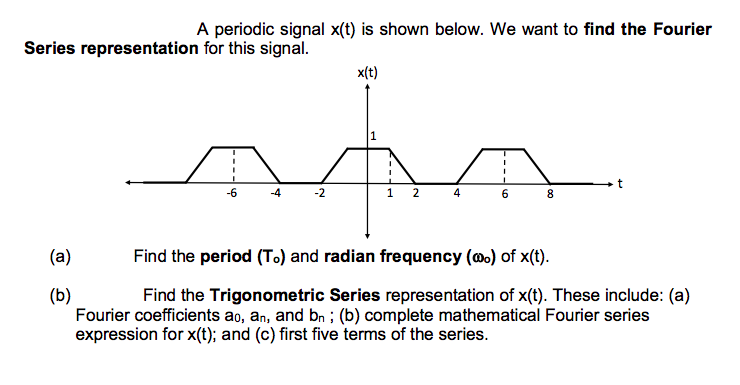A periodic signal x(t) is shown below. We want to find the Fourier Series representation for this signal. x(t) AA -4 -2 1 2 4 6 8 (a) Find the period (T.) and radian frequency (wo) of (t). (b) Find the Trigonometric Series representation of X(t). These include: (a) Fourier coefficients ao, an, and bn ; (b) complete mathematical Fourier series expression for X(t); and (c) first five terms of the series.

• ### Problem 32: (20 points) Consider a periodic signal f(t), with fundamental period To, that has the...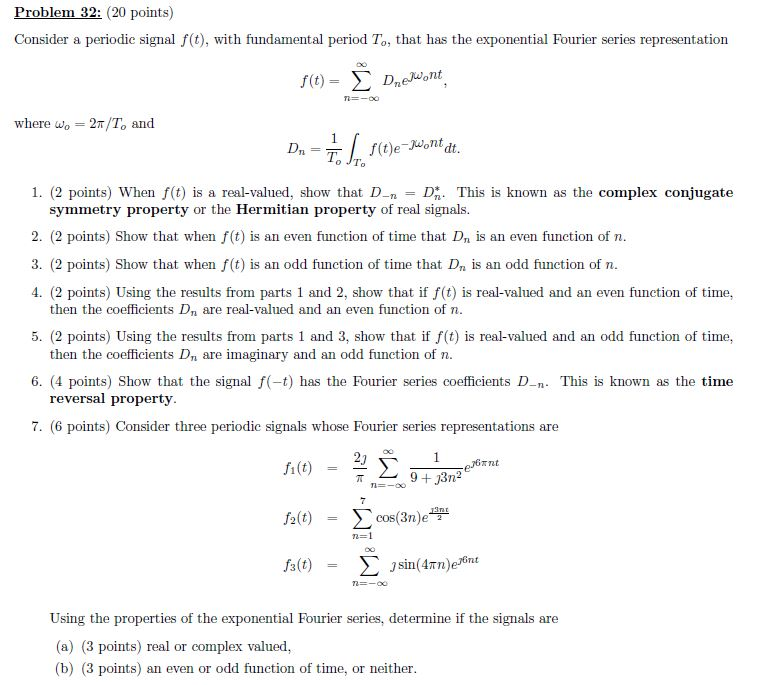Problem 32: (20 points) Consider a periodic signal f(t), with fundamental period To, that has the exponential Fourier series representation f(t) = Σ Dnejuont . where wo 2T/To and 1. (2 points) When f(t) is a real-valued, show that DD This is known as the complex conjugate symmetry property or the Hermitian property of real signals. 2. (2 points) Show that when f(t) is an even function of time that Dn is an even function of n 3. (2 points)...

• ### Question 3 (30 points) Consider the signals defined below: *:(t) = cos(2) xz(t) = cos(4+) a)...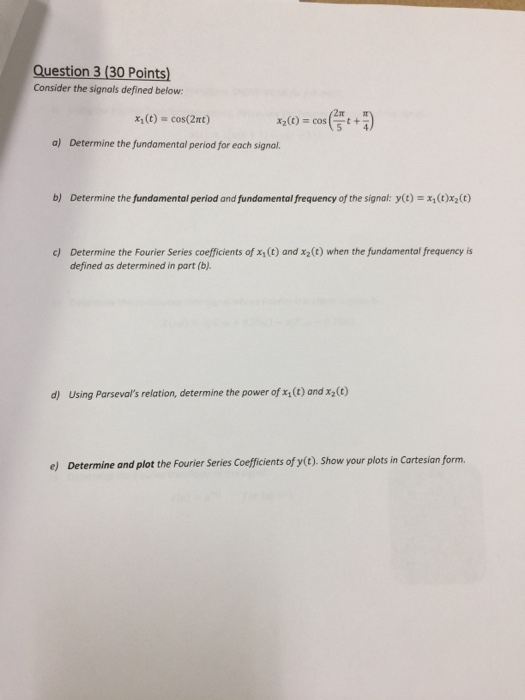Question 3 (30 points) Consider the signals defined below: *:(t) = cos(2) xz(t) = cos(4+) a) Determine the fundamental period for each signal. b) Determine the fundamental period and fundamental frequency of the signal: y(t) = x;(C)x(0) (t) and x2(c) when the fundamental frequency is c) Determine the Fourier Series coefficients of defined as determined in part (b). d) Using Parseval's relation, determine the power of xy(t) and xy(t) e) Determine and plot the Fourier Series Coefficients of y(t). Show...

• ### Problem 1 (20 pts) Suppose that x(t) = e 2 for 0 st <3 and is...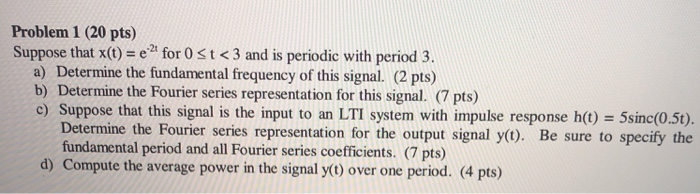Problem 1 (20 pts) Suppose that x(t) = e 2 for 0 st <3 and is periodic with period 3. a) Determine the fundamental frequency of this signal. (2 pts) b) Determine the Fourier series representation for this signal. (7 pts) c) Suppose that this signal is the input to an LTI system with impulse response h(t) = 5sinc(0.5t). Determine the Fourier series representation for the output signal y(t). Be sure to specify the fundamental period and all Fourier series...

• ### (b) Let X(ju) denote the Fourier transform of the signal r(t) shown in the figure x(t) 2 -2 1 2 Using the properties of the Fourier transform (and without explicitly evaluating X(jw)), ii. (5 pts)...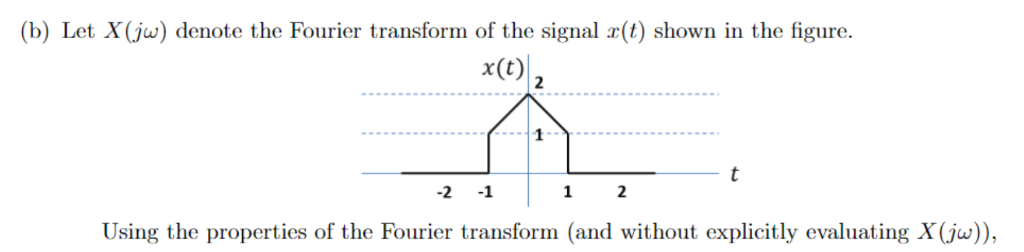(b) Let X(ju) denote the Fourier transform of the signal r(t) shown in the figure x(t) 2 -2 1 2 Using the properties of the Fourier transform (and without explicitly evaluating X(jw)), ii. (5 pts) Find2X(jw)dw. Hint: Apply the definition of the inverse Fourier transform formula, and you can also recall the time shift property for Fourier Transform. (c) (5 pts) Fourier Series. Consider the periodic signal r(t) below: 1 x(t) 1 -2 ·1/4 Transform r(t) into its Fourier Series...

• ### Problem (3) a) A periodic square wave signal x(t) is shown below, it is required to answer the below questions: x(t) 1. What is the period and the duration of such a signal? 2. Determine the fund...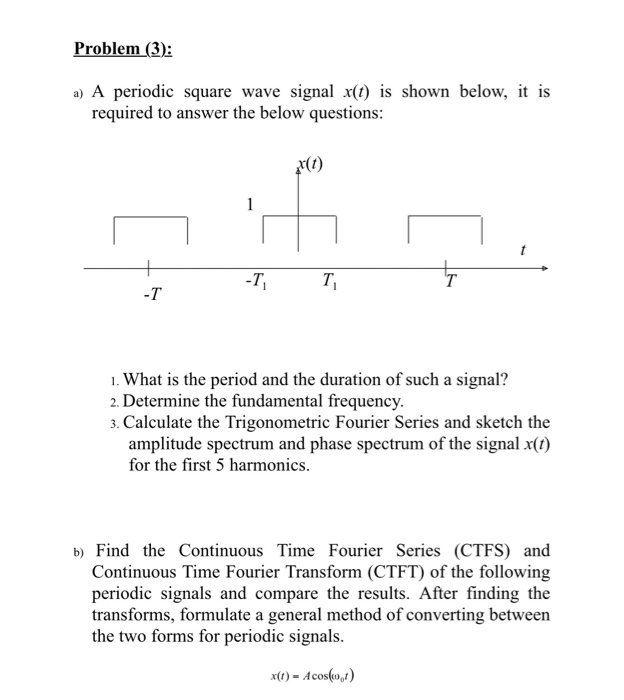Problem (3) a) A periodic square wave signal x(t) is shown below, it is required to answer the below questions: x(t) 1. What is the period and the duration of such a signal? 2. Determine the fundamental frequency. 3. Calculate the Trigonometric Fourier Series and sketch the amplitude spectrum and phase spectrum of the signal x(t) for the first 5 harmonics. b) Find the Continuous Time Fourier Series (CTFS) and Continuous Time Fourier Transform (CTFT) of the following periodic signals...

Free Homework App